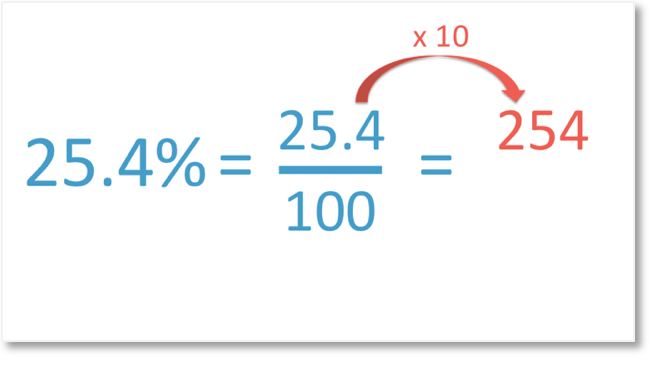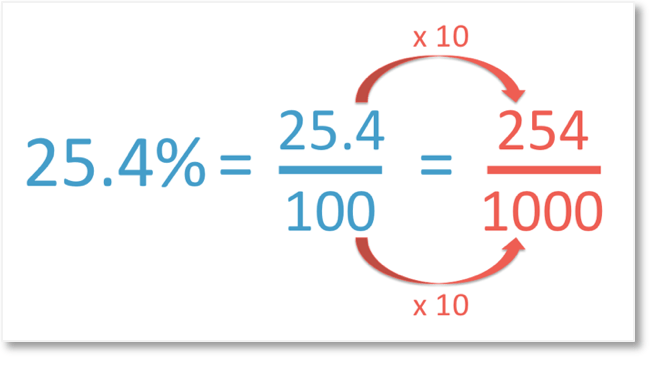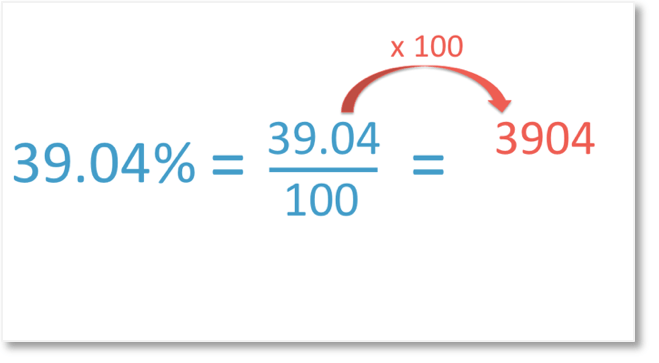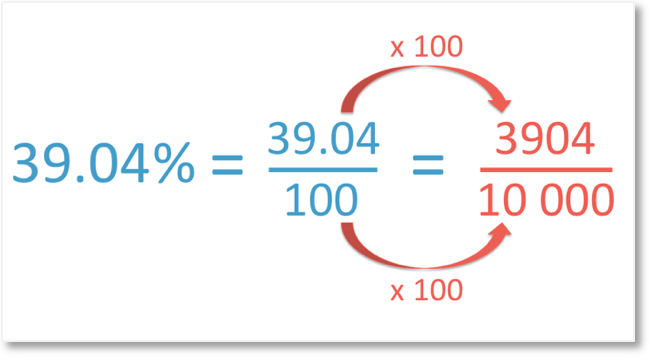# How to Convert Decimal Percentages to Fractions

How to Convert Decimal Percentages to Fractions

Supporting Lessons

## How do we Convert Decimal Percentages to Fractions

Percent means out of 100.

Per means out of and cent means 100.

Below, we have 25.4%.Percent means out of 100. So, we will have a fraction out of 100.We have 25.4%. So, we have 25.4 out of 100.

We don’t write decimals in proper fractions.

We will therefore convert to an equivalent fraction where both the numerator and denominator are whole numbers.The easiest way to do this is to move all of the digits in our decimal 25.4 one place to the left.

To do this, we multiply by 10.

25.4 x 10 = 254

The numerator of our fraction will therefore be 254.

Because we multiplied the numerator by 10, we must also multiply the denominator by 10.100 x 10 = 1000

Therefore,

25.4% = 254/1000 .

Below, we have the decimal percentage of 39.04%.Percent means out of 100. So, we have 39.04 out of 100.

We don’t want any decimals in our fractions.

We will therefore find an equivalent fraction where both the numerator and denominator are whole numbers.To convert 39.04 percent to a fraction, we begin by moving all of the digits two places to the left.

We can do this by multiplying by 100.

39.04 x 100 = 3904

Because we multiplied the numerator by 100, we must also multiply the denominator by 100.100 x 100 = 10 000

Therefore,

39.04% = 3904/10 000.